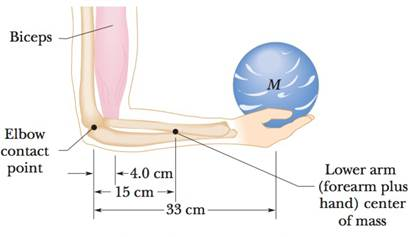Fundamentals of Physics Extended
10th Edition
ISBN: 9781118230725
Author: David Halliday, Robert Resnick, Jearl Walker
Publisher: Wiley, John & Sons, Incorporated#### Concept explainers#### Videos

Textbook QuestionChapter 12, Problem 20P

A bowler holds a bowling ball (M = 7.2 kg) in the palm of his hand (Fig. 12-37). His upper arm is vertical; his lower arm (1.8 kg) is horizontal. What is the magnitude of (a) the force of the biceps muscle on the lower arm and (b) the force between the bony structures at the elbow contact point?Figure 12-37 Problem 20.07:17
Students have asked these similar questions
a vertical uniform beam of length Lthat is hinged at its lower end.A horizontal force F is applied to the beam at distance y from the lower end. The beam remainsvertical because of a cable attached at the upper end, at angle uwith the horizontal. gives the tension T in the cableas a function of the position of the applied force given as a fractiony/L of the beam length.The scale of the T axis is set by Ts= 600 N.Figure 12-49c gives the magnitude Fh of the horizontal force on thebeam from the hinge, also as a function of y/L. Evaluate (a) angle uand (b) the magnitude of .
One end of a uniform rod of weight w = 73.5 N and length L = 2.55 m is supported by a cable at an angle of ? = 37.0°above the rod. The other end rests on a small frictionless support and presses into a wall as shown in the figure. Determine the magnitude n of the vertical normal force exerted by the support on the rod and find the magnitude T of the tension in the cable.
Two ladders, 4.00 m and 3.00 m long, are hinged at point A and tied together by a horizontal rope 0.90 m above the floor. The ladders weigh 480 N and 360 N, respectively, and the center of gravity of each is at its center. Assume that the floor is freshly waxed and frictionless. (a) Find the upward force at the bottom of each ladder. (b) Find the tension in the rope. (c) Find the magnitude of the force one ladder exerts on the other at point A. (d) If an 800-N painter stands at point A, find the tension in the horizontal rope.